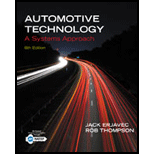Chapter 3, Problem 9RQAutomotive Technology: A Systems A...

6th Edition
Jack Erjavec + 1 other
ISBN: 9781133612315

Solutions

Chapter
SectionAutomotive Technology: A Systems A...

6th Edition
Jack Erjavec + 1 other
ISBN: 9781133612315
Textbook Problem

Describe the effect of pressure on an enclosed volume of a gas.

To determine

The effect of pressure on an enclosed volume of a gas.

Explanation

Given Information:

Volume is constant.

Let take gas equation.

PV = nRT

Now there are three parameters are constant volume, number of moles, ideal gas constant. So,the pressure is directly proportional to the temperature...

Still sussing out bartleby?

Check out a sample textbook solution.

See a sample solution

The Solution to Your Study Problems

Bartleby provides explanations to thousands of textbook problems written by our experts, many with advanced degrees!

Get Started